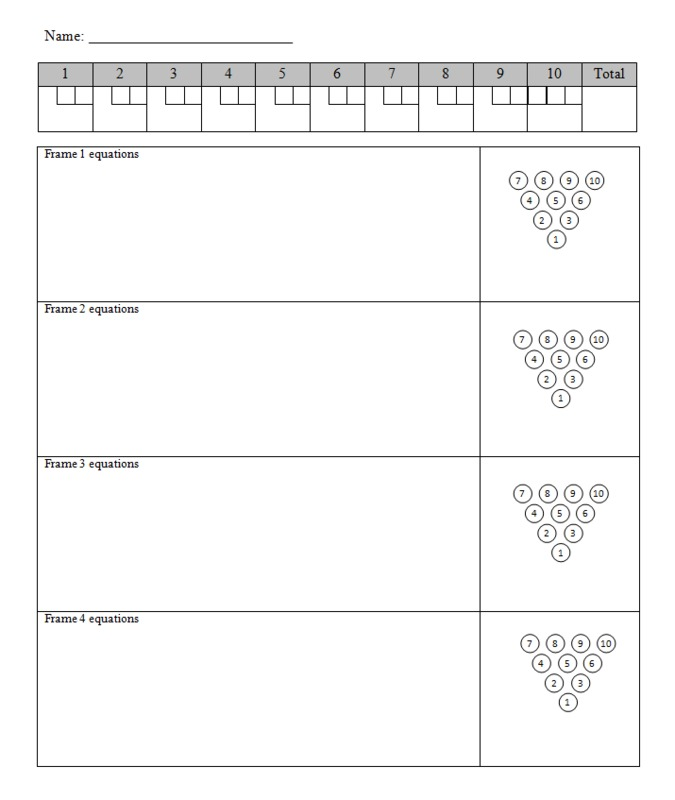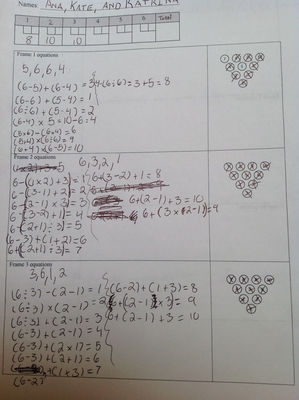# Bowling for Numbers

Alignments to Content Standards: 5.OA.A.1

#### Materials:

• 4 dice per team
• Recording sheet
• Two-minute timer for each turn

#### Action:

Have students work in groups of 2 - 4. Introduce the game with an example, and then have them play independently. Discussion of "challenging rolls" afterwards can be productive.

Students roll the 4 dice to generate their seed numbers. They then use those 4 numbers to create as many numbers as they can (1 - 10). Scoring is done as in bowling; numbered "pins" are "knocked down" by creating an expression equal to the number.

The game can be structured in two different ways to assure that students are checking each other's expressions and verifying that they are written as intended:

1. During a student's turn, have them record just the expressions (not the intended result), and then pass the set to another student (a judge). That judge then computes each expression as written and records which pins were knocked down.

2. Have the students play in teams. Each team tries to achieve a "strike" (knocking down all of the pins, which is almost always possible). Striving for the strike encourages students to brainstorm strategies for the "difficult" numbers, which leads them to discuss parts of each expression they have created already.## IM Commentary

The purpose of this game is to help students think flexibly about numbers and operations and to record multiple operations using proper notation.

Students eager to knock down all of the pins quickly develop patterns in their expressions. They may re-use parts of an expression, perhaps changing just the final operation; for example, if the dice showed 1, 2, 5, 5, they might write:

$(2 + 1) + (5 \div 5) = 4$

$(2 + 1) - (5 \div 5) = 2$

$(2 + 1) \times (5 \div 5) = 3$

Or they might change one of the internal operations:

$(2 + 1) \times (5 \div 5) = 3$

$(2 - 1) \times (5 \div 5) = 1$

$(2 \times 1) \times (5 \div 5) = 2$

Similar-but-different expressions like these emphasize the importance of parentheses and the flexibility they give us in creating expressions with very specific meanings.

## Solution

Here is an example of a student worksheet that has been filled out: